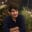Related Tags

general
communitycreator

# Float vs. doubleMuhammad Adan

The main difference between double and float, both of which are floating-point datatypes, is precision.

Double, as the name suggests, is double the precision of float. Where a float is represented by 32 bits, a double is represented by 64 bits.

This leads to a difference in storage space requirement. Float data-type requires 4 Bytes for storage; whereas, a double requires 8 Bytes of storage.

## IEEE 754 Format

The IEEE format for floating-point representation is a standard format across multiple hardware floating-point units.

A float is a 32-bit single-precision Floating Point Number in the IEEE 754where the Most Significant Bit MSB is reserved as a sign bit, the next 8 bits are used for the exponent, and the last 23 bits for the value. This leads to a precision of 6-7 decimal digits. The range of numbers it can represent is $2^{-126}$ to $2^{127}$.

In contrast, a double is a 64-bit double-precision Floating Point Number according to IEEE. The MSB is reserved as a sign bit, the next 11 bits for the exponent, and the last 52 bits for the value, which results in a precision of 15-16 decimal digits. The range of numbers it can represent is $2^{-1022}$ to $2^{1023}$.

## When to use?

A float data type is typically used when we are concerned about the high demands of processing power. One such case would be graphics libraries such as DirectX, Vulkan, OpenGL, etc. Video games typically use single-precision floating points.

The float data type is also used in cases where we can tolerate rounding errors.

A double data type is used in cases of highly scientific simulations where highly sophisticated numerical methods are used that require precise calculations.

#include <iostream>
using namespace std;

int main() {
// Creating a double type variable
double d = 3.912348239293;

// Creating a float type variable
float f = 3.912348239293f;

// Printing the two variables
cout << "Double = " << d << endl;
cout << "Float = " << f << endl;
return 0;
}

RELATED TAGS

general
communitycreator

CONTRIBUTORMuhammad Adan
RELATED COURSES

View all Courses

Keep Exploring

Learn in-demand tech skills in half the time